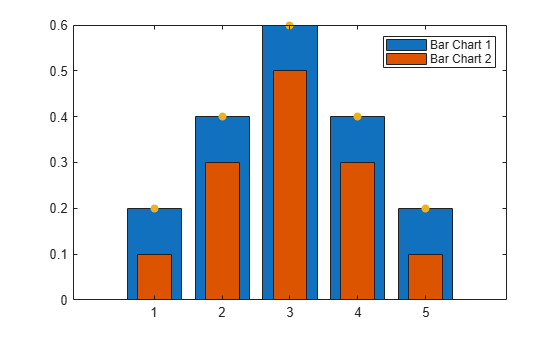Legends are a useful way to label data series plotted on a graph. These examples show how to create a legend and make some common modifications, such as changing the location, setting the font size, and adding a title. You also can create a legend with multiple columns or create a legend for a subset of the plotted data.

### Create Simple Legend

Create a figure with a line chart and a scatter chart. Add a legend with a description for each chart. Specify the legend labels as inputs to the `legend` function.

```figure x1 = linspace(0,5); y1 = sin(x1/2); plot(x1,y1) hold on x2 = [0 1 2 3 4 5]; y2 = [0.2 0.3 0.6 1 0.7 0.6]; scatter(x2,y2,'filled') hold off legend('sin(x/2)','2016')```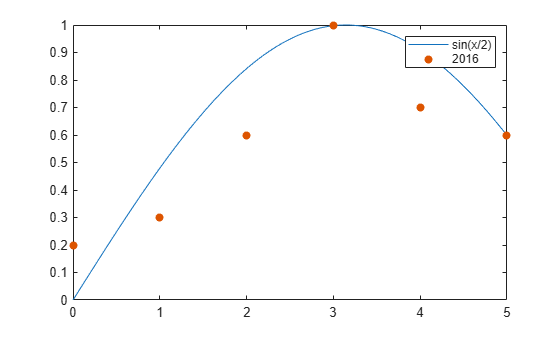### Specify Labels Using DisplayName

Alternatively, you can specify the legend labels using the `DisplayName` property. Set the `DisplayName` property as a name-value pair when calling the plotting functions. Then, call the `legend` command to create the legend.

```x1 = linspace(0,5); y1 = sin(x1/2); plot(x1,y1,'DisplayName','sin(x/2)') hold on x2 = [0 1 2 3 4 5]; y2 = [0.2 0.3 0.6 1 0.7 0.6]; scatter(x2,y2,'filled','DisplayName','2016') legend```

Legends automatically update when you add or delete a data series. If you add more data to the axes, use the `DisplayName` property to specify the labels. If you do not set the `DisplayName` property, then the legend uses a label of the form `'dataN'`.

Add a scatter chart for 2017 data.

```x3 = [0 1 2 3 4 5]; y3 = [0.1 0.4 0.6 0.9 0.8 0.7]; scatter(x3,y3,'filled','DisplayName','2017') drawnow hold off```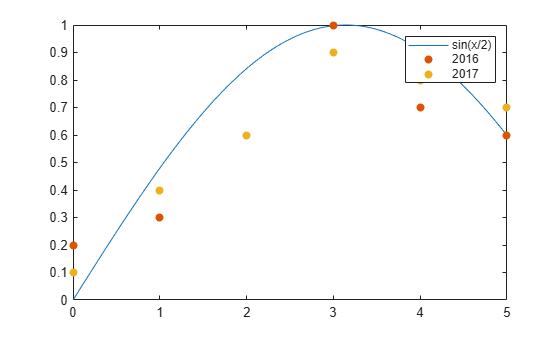### Customize Legend Appearance

The `legend` function creates a `Legend` object. L`egend` objects have properties that you can use to customize the appearance of the legend, such as the `Location`, `Orientation`, `FontSize`, and `Title` properties. For a full list, see Legend Properties.

You can set properties in two ways:

• Use name-value pairs in the `legend` command. In most cases, when you use name-value pairs, you must specify the labels in a cell array, such as `legend({'label1','label2'},'FontSize',14)`.

• Use the `Legend` object. You can return the `Legend` object as an output argument from the `legend` function, such as `lgd = legend`. Then, use `lgd` with dot notation to set properties, such as `lgd.FontSize = 14`.

### Legend Location and Orientation

Specify the legend location and orientation by setting the `Location` and `Orientation` properties as name-value pairs. Set the location to one of the eight cardinal or intercardinal directions, in this case, `'northwest'`. Set the orientation to `'vertical'` (the default) or `'horizontal'`, as in this case. Specify the labels in a cell array.

```x1 = linspace(0,5); y1 = sin(x1/2); plot(x1,y1) hold on x2 = [0 1 2 3 4 5]; y2 = [0.2 0.3 0.6 1 0.7 0.6]; scatter(x2,y2,'filled') hold off legend({'sin(x/2)','2016'},'Location','northwest','Orientation','horizontal')```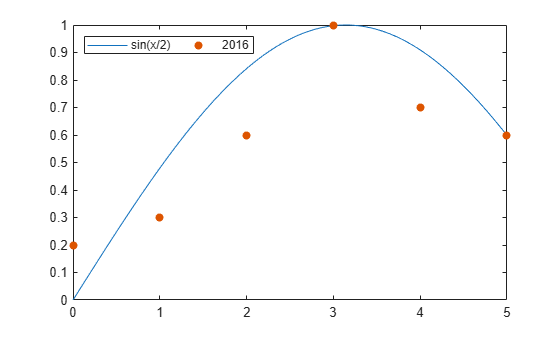### Legend Font Size and Title

Specify the legend font size and title by setting the `FontSize` and `Title` properties. Assign the `Legend` object to the variable `lgd`. Then, use `lgd` to change the properties using dot notation.

```x1 = linspace(0,5); y1 = sin(x1/2); plot(x1,y1,'DisplayName','sin(x/2)') hold on x2 = [0 1 2 3 4 5]; y2 = [0.2 0.3 0.6 1 0.7 0.6]; scatter(x2,y2,'filled','DisplayName','2016') hold off lgd = legend; lgd.FontSize = 14; lgd.Title.String = '2016 Data';```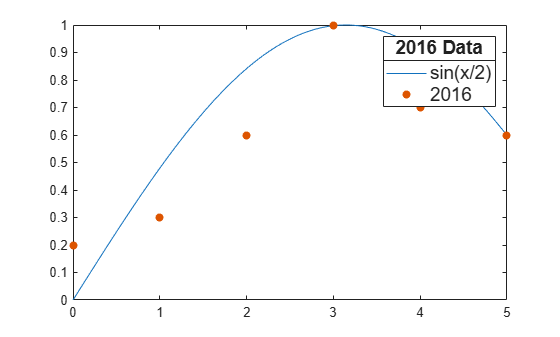### Legend with Multiple Columns

Create a chart with six line plots. Add a legend with two columns by setting the `NumColumns` property to 2.

```x = linspace(0,10); y1 = sin(x); y2 = sin(0.9*x); y3 = sin(0.8*x); y4 = sin(0.7*x); y5 = sin(0.6*x); y6 = sin(0.5*x); plot(x,y1,'DisplayName','sin(x)') hold on plot(x,y2,'DisplayName','sin(0.9x)') plot(x,y3,'DisplayName','sin(0.8x)') plot(x,y4,'DisplayName','sin(0.7x)') plot(x,y5,'DisplayName','sin(0.6x)') plot(x,y6,'DisplayName','sin(0.5x)') hold off lgd = legend; lgd.NumColumns = 2;```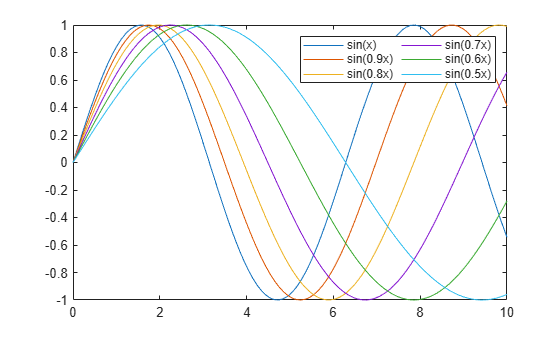### Include Subset of Charts in Legend

Combine two bar charts and a scatter chart. Create a legend that includes only the bar charts by specifying the `Bar` objects, `b1` and `b2`, as the first input argument to the `legend` function. Specify the objects in a vector.

```x = [1 2 3 4 5]; y1 = [.2 .4 .6 .4 .2]; b1 = bar(x,y1); hold on y2 = [.1 .3 .5 .3 .1]; b2 = bar(x,y2,'BarWidth',0.5); y3 = [.2 .4 .6 .4 .2]; s = scatter(x,y3,'filled'); hold off legend([b1 b2],'Bar Chart 1','Bar Chart 2')```